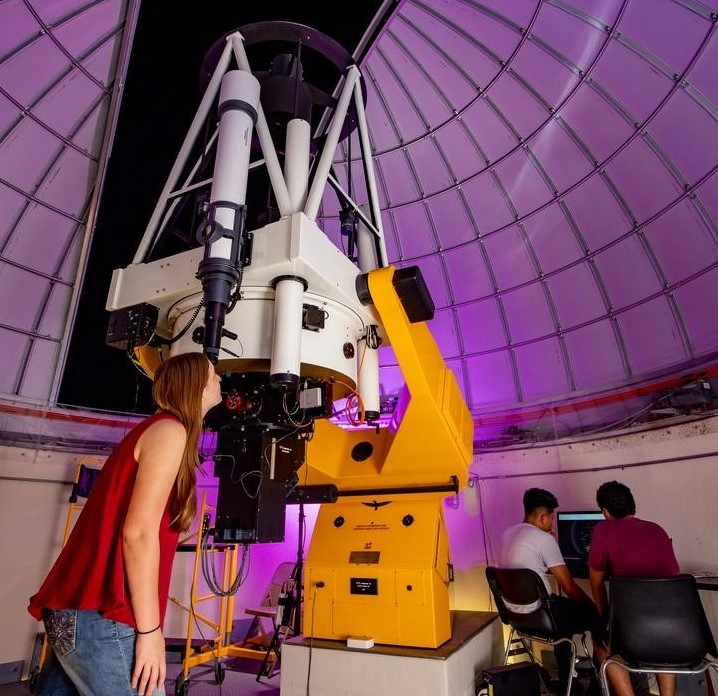## Aerospace, Physics, and Space Science Faculty Publications

### Perturbations of gravitational instantons

Article

#### Publication Title

Physical Review D.

#### Abstract

Ashtekar's spinorial formulation of general relativity is used to study perturbations of gravitational instantons corresponding to finite-action solutions of the Euclidean Einstein equations (with a nonzero cosmological constant) possessing an anti-self-dual Weyl curvature tensor. It is shown that, with an appropriate "on-shell" form of infinitesimal gauge transformations, the space of solutions to the can be described in terms of an elliptic complex; the cohomology of the complex defines gauge-inequivalent perturbations. Using this elliptic complex we prove that there are no nontrivial solutions to the linearized instanton equation on conformally anti-self-dual Einstein spaces with a positive cosmological constant. Thus, the space of gravitational instantons is discrete when the cosmological constant is positive; i.e., the dimension of the gravitational moduli space in this case is zero. We discuss the issue of linearization stability as well as the feasibility of using the to compute the dimension of the gravitational moduli space when the cosmological constant is negative. © 1990 The American Physical Society.

3620

3627

#### DOI

10.1103/PhysRevD.41.3620

1-15-1990

COinS